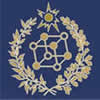Phys.Faculty Site

Some Presentations (in Russian and English)Memorial E.A.Kaner Seminar

Materials for Study

 Theoretical Mechanics [ru] 2. SEMINARS AND HOME TASKS Electrodynamics 1.Course Content 2. Home Tasks 3. Test Examples (3rd year, Fall Term) 4. Midterm Exam Problem Examples (3rd year Spring Term) 5. Final Exam Problems (3rd year Spring Term) Quantum Mechanics. (School of Physics) 1. Course Content 2. Home Tasks 3. Some Lecture Notes [ru] 4."Quantum Dictation" Questions 5. Individual Tasks to Solve.(3rd year, Spring Term) Example of Ind.Task 6. Individual Tasks to Solve [ru] (4rd year, Fall Term) 7."Quantum Minimum Formulas" 8. Final Exam Test Examples [ru] 7. SEMINARS AND HOME TASKS 3d year, spring term 1. Mathematical tools of quantum mechanics: Linear algebra, theory of linear space, theory of linear operators. 2. Mathematical tools of quantum mechanics: operations with operators. Finding eigenfunctions and eigenvalues of Hermitian and non-Hermitian operators. Functions from operators. 3. Mathematical tools of quantum mechanics: Operator function (continuation). Matrix-operators in space En. Properties of Pauli matrices. 4. Mathematical tools of quantum mechanics: Calculating expectation value of operators. Elements of representation theory. Discrete and continuous representations. 5. Mathematical tools of quantum mechanics: Momentum representation. One-dimensional (1D )movement: 1D free particle, 1D infinite square well. 6. 1D movement: One-dimensional movement in the piecewise continuous potentials. Discrete spectrum and continuous spectrum. 7. 1D movement: Movement in the piecewise continuous potentials. (continuation). Schrodinger’s equation in momentum representation. Transfer-matrix: calculating of transmission coefficient, energy spectrum of periodic potentials. 4th year, fall term 8-9. Refresher course of previous semester:main equations and concepts of quantum mechanics. New topic: Angular Momentum. Commutation relations. Eigenfunctions and eigenvalues of of the angular momentum z-projection and the squared angular momentum. "Ladder operators". 10. Central field: two-body problem in QM, plane rigid rotator, spacial rigid rotator. 11. Central field: spherical waves, spherically symmetrical rectangular well of finite depth. 12. Perturbation Theory (PT): time-independent PT. 13. Perturbation Theory (PT)): time-dependent PT. 14. Quasi-classical approximation: Bohr-Sommerfeld quantization rule. 15. Quasi-classical approximation: tunneling. 16. Spin-1/2. 17. Spin.(ending) Quantum Mechanics. (School of Radiophysics)[ru] 1. Course Content. [ukr] 2. Seminars 2017 [ru] 3. Lecture Notes ([ukr] Fall Term 2017) 4. Evaluation criteria 2017. [ru] 5."Quantum minimum." [en] Statistical Physics [ru] 1. Course content 2. List of practical exercises 3. Tasks for practical lessons 4. Midterm tasks examples 5. Final Exam 6. Topics for practical lessons: 1 , 2 , 3 , 4 , 5 , 6 , 7 , 8 , 9 , 10, 11, 12 7. Quantum Statistics Lecture Notes. Physical Kinetics [ru] Course Content

Some Lesture's Presentations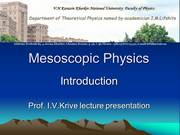Mesoscopic Physics and Fundamentals of Nanotechnology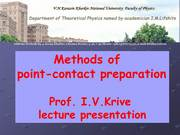Mesoscopic Physics and Fundamentals of NanotechnologyMesoscopic Physics and Fundamentals of NanotechnologyTheory of Magnetism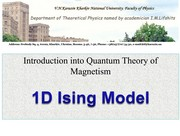Theory of Magnetism1D Ising Model [ru]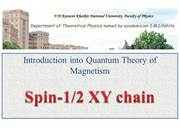Theory of Magnetism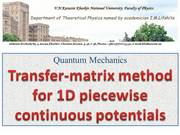Quantum Mechanics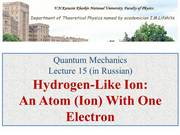Quantum Mechanics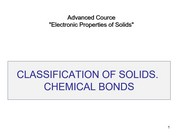Solid State Physics [ru]

Designed by E.V.Ezerskaya  © 2012